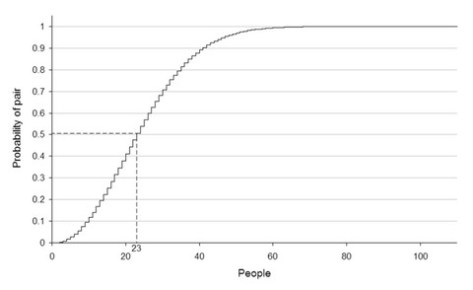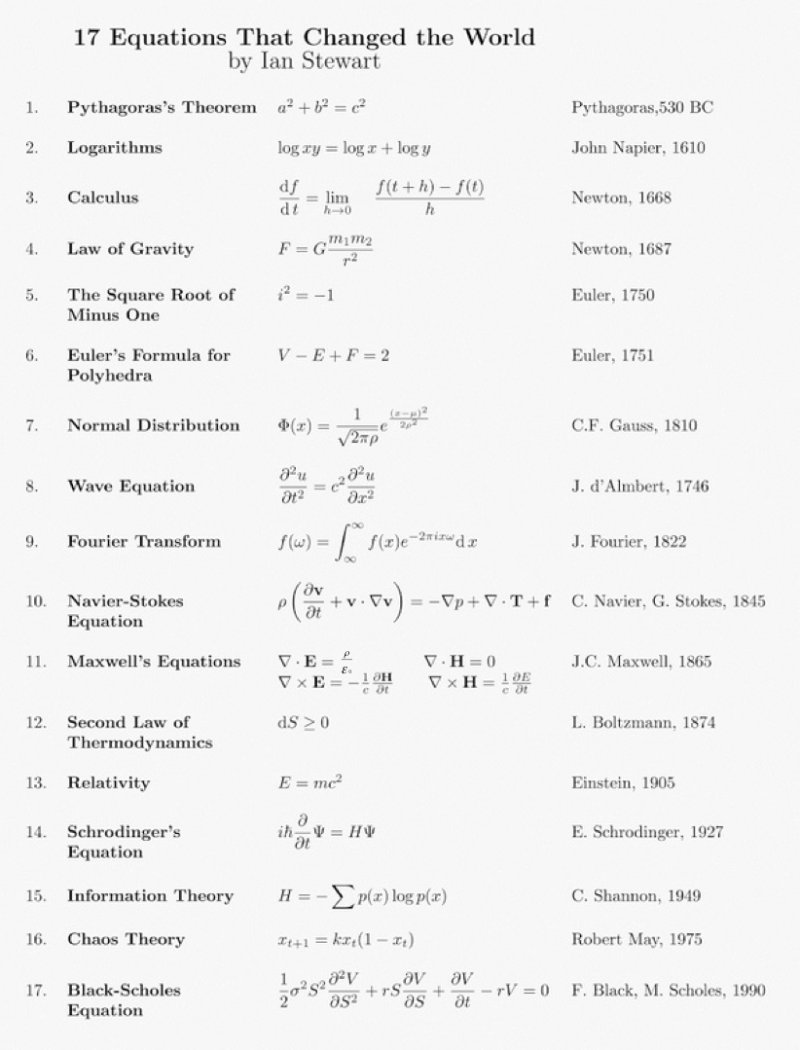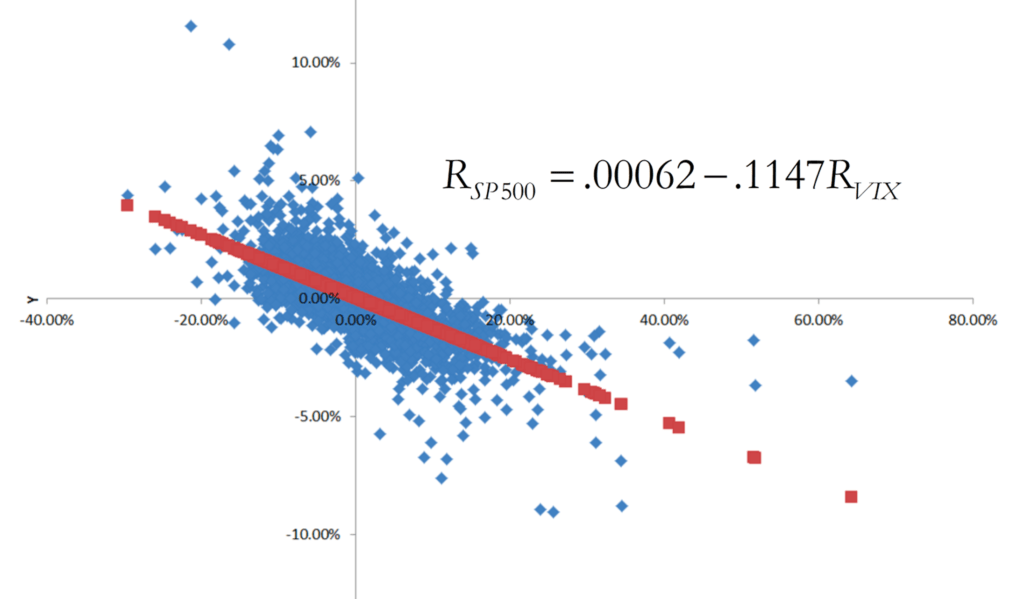## The Birthday Paradox: an interesting probability problem involving “statistically independent” events

During this week’s statistics tutorials, we discussed (among other things) the concept of statistical independence, and focused attention on some important implications of statistical independence for probability distributions such as the binomial and normal distributions.

Here, I’d like to call everyone’s attention to an interesting (non-finance) probability problem related to statistical independence. Specifically, consider the so-called “Birthday Paradox”. The Birthday Paradox pertains to the probability that in a set of randomly chosen people, some pair of them will have the same birthday. Counter-intuitively, in a group of 23 randomly chosen people, there is slightly more than a 50% probability that some pair of them will both have been born on the same day.

To compute the probability that two people in a group of n people have the same birthday, we disregard variations in the distribution, such as leap years, twins, seasonal or weekday variations, and assume that the 365 possible birthdays are equally likely. Thus, we assume that birthdates are statistically independent events. Consequently, the probability of two randomly chosen people not sharing the same birthday is 364/365. According to the combinatorial equation, the number of unique pairs in a group of n people is n!/2!(n-2)! = n(n-1)/2. Assuming a uniform distribution (i.e., that all dates are equally probable), this means that the probability that no pair in a group of n people shares the same birthday is equal to p(n) = (364/365)^[n(n-1)/2]. The event of at least two of the n persons having the same birthday is complementary to all n birthdays being different. Therefore, its probability is p’(n) = 1 – (364/365)^[n(n-1)/2].

Given these assumptions, suppose that we are interested in determining how many randomly chosen people are needed in order for there to be a 50% probability that at least two persons share the same birthday. In other words, we are interested in finding the value of n which causes p(n) to equal 0.50. Therefore, 0.50 = (364/365)^[n(n-1)/2]; taking natural logs of both sides and rearranging, we obtain (ln 0.50)/(ln 364/365) = n(n-1)/2. Solving for n, we obtain 505.304 = n(n -1); therefore, n is approximately equal to 23.

The following graph illustrates how the probability that a pair of people share the same birthday varies as the number of people in the sample increases:It is worthwhile noting that real-life birthday distributions are not uniform since not all dates are equally likely. For example, in the Northern Hemisphere, many children are born in the summer, especially during the months of August and September. In the United States, many children are conceived around the holidays of Christmas and New Year’s Day. Also, because hospitals rarely schedule C-sections and induced labor on the weekend, more Americans are born on Mondays and Tuesdays than on weekends; where many people share a birth year (e.g., a class in a school), this creates a tendency toward particular dates. Both of these factors tend to increase the chance of identical birthdates since a denser subset has more possible pairs (in the extreme case when everyone was born on three days of the week, there would obviously be many identical birthdays!).

 Note that since 15 students are enrolled in Finance 4366 this semester, the probability that at least two Finance 4366 students share the same birthday is p’(15) = 1 – (364/365)^[15(14)/2] = 25%; this probability can also be inferred from the above figure.

## On the ancient origin of the word “algorithm”

The January 24th assigned reading entitled “The New Religion of Risk Management” (by Peter Bernstein, March-April 1996 issue of Harvard Business Review) provides a succinct synopsis of the same author’s 1996 book entitled “Against the Gods: The Remarkable Story of Risk“. Here’s a fascinating quote from page 33 of “Against the Gods” which explains the ancient origin of the word “algorithm”:

“The earliest known work in Arabic arithmetic was written by al­Khowarizmi, a mathematician who lived around 825, some four hun­dred years before Fibonacci. Although few beneficiaries of his work are likely to have heard of him, most of us know of him indirectly. Try saying “al­Khowarizmi” fast. That’s where we get the word “algo­rithm,” which means rules for computing.”

Note: The book cover shown above is a copy of a 1633 oil-on-canvas painting by the Dutch Golden Age painter Rembrandt van Rijn.

## The 17 equations that changed the course of history (spoiler alert: we use 4 of these equations in Finance 4366!)

I especially like the fact that Ian Stewart includes the famous Black-Scholes equation (equation #17) on his list of the 17 equations that changed the course of history; Equations (2), (3), (7), and (17) play particularly important roles in Finance 4366!From Ian Stewart’s book, these 17 math equations changed the course of human history.

## Origin of the “Product Rule”, and Visualizing Taylor polynomial approximations

This blog entry provides a helpful follow-up for a couple of calculus-related topics that we covered during today’s Mathematics Tutorial.

1. See page 12 of the above-referenced lecture note.  There, the equation for a parabola ($y = {x^2}$) appears, and the claim that$\frac{{dy}}{{dx}} = 2x$ is corroborated by solving the following expression:In the 11-minute Khan Academy video at https://youtu.be/HEH_oKNLgUU, Sal Kahn takes on the solution of this problem in a very succinct and easy-to-comprehend fashion.
2. In his video lesson entitled “Visualizing Taylor polynomial approximations”, Sal Kahn replicates the tail end of today’s Finance 4366 class meeting in which we approximated y = ex with a Taylor polynomial centered at x=0 (as also shown in pp. 18-23 of the Mathematics Tutorial lecture note).  Sal approximates y = ex with a Taylor polynomial centered at x=3 instead of x=0, but the same insight obtains in both cases, which is that the accuracy of Taylor polynomial approximations increases as the order of the polynomial increases.

## Calculus, Probability and Statistics, and a preview of future topics in Finance 4366

Calculus (yesterday’s Finance 4366 class topic) and probability and statistics (next week’s Finance 4366 class topics) are foundational for the theory of pricing and managing risk with financial derivatives.

On Tuesday, January 31, we will introduce and describe the nature of financial derivatives, and motivate their study with examples of forwards, futures, and options. Derivatives are so named because they derive their values from one or more underlying assets. Underlying assets typically involve traded financial assets such as stocks, bonds, currencies, or other derivatives, but derivatives can derive value from pretty much anything. For example, the Chicago Mercantile Exchange (CME) offers exchange-traded weather futures and options contracts (see “Market Futures: Introduction To Weather Derivatives“). There are also so-called “prediction” markets in which derivatives based upon the outcome of political events are actively traded (see “Prediction Market“).

Besides introducing financial derivatives and discussing various institutional aspects of markets in which they are traded, we’ll consider various properties of forward and option contracts, since virtually all financial derivatives feature payoffs that are isomorphic to either or both schemes. For example, a futures contract is simply an exchange-traded version of a forward contract. Similarly, since swaps involve exchanges between counter-parties of payment streams over time, these instruments essentially represent a series of forward contracts. In the option space, besides traded stock options, many corporate securities feature “embedded” options; e.g., a convertible bond represents a combination of a non-convertible bond plus a call option on company stock. Similarly, when a company makes an investment, so-called “real” options to expand or abandon the investment at some future is often present.

Perhaps the most important (pre-Midterm 1) idea that we’ll introduce is the concept of a so-called “arbitrage-free” price for a financial derivative. While details will follow, the basic idea is that one can replicate the payoffs on a forward or option by forming a portfolio comprising the underlying asset and a riskless bond. This portfolio is called the “replicating” portfolio, since, by design, it replicates the payoffs on the forward or option. Since the forward or option and its replicating portfolio produce the same payoffs, then they must also have the same value. However, suppose the replicating portfolio (forward or option) is more expensive than the forward or option (replicating portfolio). If this occurs, then one can earn a riskless arbitrage profit by simply selling the replicating portfolio (forward or option) and buying the forward or option (replicating portfolio). However, competition will ensure that opportunities for riskless arbitrage profits vanish quickly. Thus the forward or option will be priced such that one cannot earn arbitrage profit from playing this game.

## On the relationship between the S&P 500 and the CBOE Volatility Index (VIX)

Besides going over the course syllabus during the first day of class on Tuesday, January 17, we will also discuss a particularly important “real world” example of financial risk. Specifically, we will study the relationship between realized daily stock market returns (as measured by daily percentage changes in the SP500 stock market index) and changes in forward-looking investor expectations of stock market volatility (as indicated by daily percentage changes in the CBOE Volatility Index (VIX)):As indicated by this graph (which also appears in the lecture note for the first day of class), daily percentage changes on closing prices for the SP500 (the y-axis variable) and for the VIX (the x-axis variable) are strongly negatively correlated with each other. The blue dots are based on 8,315 contemporaneous observations of daily returns for both variables, spanning the 33-year period of time starting on January 2, 1990 and ending on December 30, 2022. When we fit a regression line through this scatter diagram, we obtain the following equation:${R_{SP500}} = .00062 - .1147{R_{VIX}}$,

where${R_{SP500}}$ corresponds to the daily return on the SP500 index and${R_{VIX}}$ corresponds to the daily return on the VIX index. The slope of this line (-0.1147) indicates that on average, daily closing SP500 returns are inversely related to daily closing VIX returns.  Furthermore, nearly half of the variation in the stock market return during this time period (specifically, 48.87%) can be statistically “explained” by changes in volatility, and the correlation between${R_{SP500}}$ and${R_{VIX}}$ came out to -0.70. While a correlation of -0.70 does not imply that daily closing values for${R_{SP500}}$ and${R_{VIX}}$ always move in opposite directions, it does suggest that this will be the case more often than not. Indeed, closing daily values recorded for${R_{SP500}}$ and${R_{VIX}}$ during this period moved inversely 78.59% of the time.